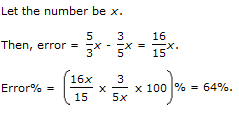6.

A student multiplied a number by 3/5 instead of

 A. 34% B. 44% C. 54% D. 64%
Explanation:7.

In an election between two candidates, one got 55% of the total valid votes, 20% of the votes were invalid. If the total number of votes was 7500, the number of valid votes that the other candidate got, was:

 A. 2700 B. 2900 C. 3000 D. 3100
Explanation: Number of valid votes = 80% of 7500 = 6000.

Valid votes polled by other candidate = 45% of 6000

= (45/100 x 6000) = 2700.

8.

Three candidates contested an election and received 1136, 7636 and 11628 votes respectively. What percentage of the total votes did the winning candidate get?

 A. 57% B. 60% C. 65% D. 90%
Explanation: Total number of votes polled = (1136 + 7636 + 11628) = 20400.

Required percentage = (11628/20400 x 100)% = 57%.

9.

Helen went to the stationers and bought things worth Euro 25, out of which 30 paise went on sales tax on taxable purchases. If the tax rate was 6%, then what was the cost of the tax free items?

 A. Euro 15 B. Euro 15.7 C. Euro 19.7 D. Euro 20
Explanation: Let the amount taxable purchases be Euro x.

Then, 6% of x = 30/100

x = (30/100 x 100/6) = 5.

Cost of tax free items = Euro [25 - (5 + 0.30)] = Euro 19.70

10.

The population of a town increased from 1,75,000 to 2,62,500 in a decade. The average percent increase of population per year is:

 A. 4.37% B. 5% C. 6% D. 8.75%